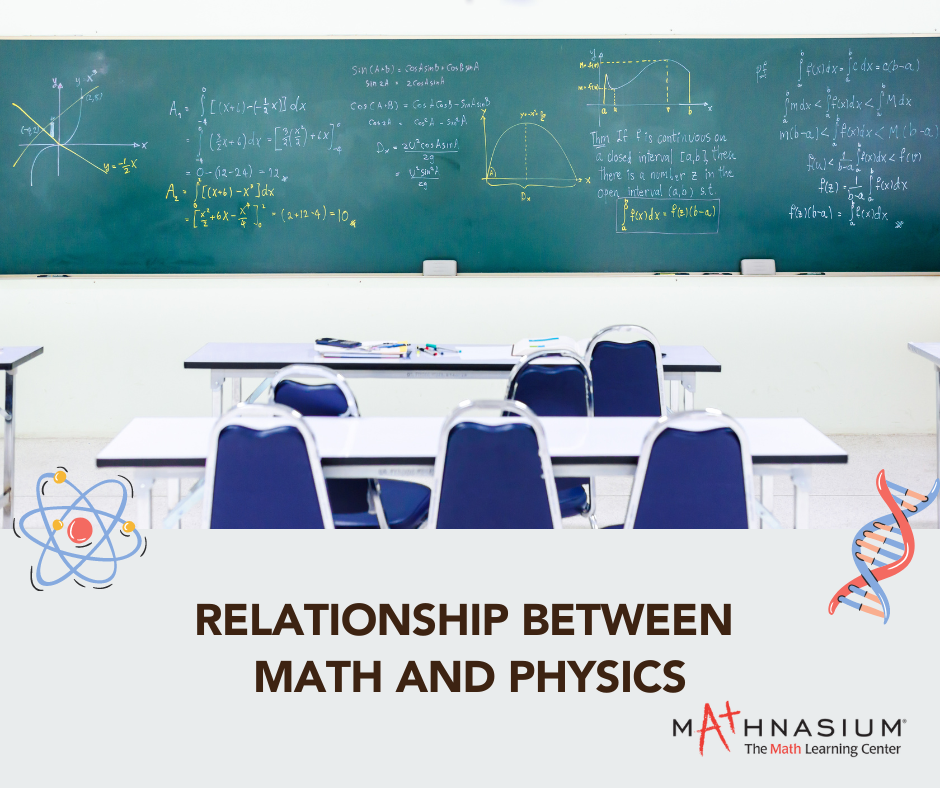877-601-6284
Get Started by Finding a Local Center

# How Math and Physics Are Connected

Nov 2, 2022 | Location Cherry Hill” Mathematics is the abstract key which turns the lock of the physical universe.” -John Polkinghorne

Students pursuing degrees in math, always have questions about why we need to study dynamics, statics, and fluids. Similarly, students in the physics field study mathematical physics, which requires them to study modern algebra and differential equations. The simple answer to this popular question is that Math and Physics are closely intertwined and connected fields. Math is a tool that physicists use to find answers to questions. For instance, Newton created calculus to describe motion. Mathematicians will also draw inspiration from theories like general relativity and quantum theory in physics to create new mathematical techniques.

Physics Without Math What if we knew about gravity but not its acceleration? That might not sound like a big deal. Still, it is the difference between just knowing that things fall and understanding how to calculate that information into ever-increasing cool and valuable applications. Mathematical information is necessary for Physics, and Physics without mathematics is useless.

Different approaches to Math and Physics Even though math and physics have a great deal in common, they adopt different approaches. Physics includes the study of both the large and small, from galaxies and planets to atoms and particles, as it is the systematic study of how matter behaves. New theories regarding the nature of the cosmos are either supported or disproved using combinations of theories, experiments, models, and observations. Math, on the other hand, focuses on abstract concepts like quantity, structure, and space. By utilizing pure logic and mathematical reasoning, mathematicians search for patterns and create new concepts and theories. Rather than relying on experiments or observations, mathematicians rely on proofs.

Mathematics has already been described as “an essential tool for physics” and physics as “a rich source of inspiration and insight in mathematics" (Edward Witten, Princeton Univ). With advances in both fields, physics and math have grown tangled over the centuries, like a complex tapestry. A well-placed set of calculational tools could be handy to physicists, or a challenging concept from physics might motivate mathematicians to develop entirely new mathematical objects or theories. Can you think of another way that the relationship between Mathematics and Physics has helped the development of fantastic inventions and technology?

##### Locations near
Could not find Center, try again